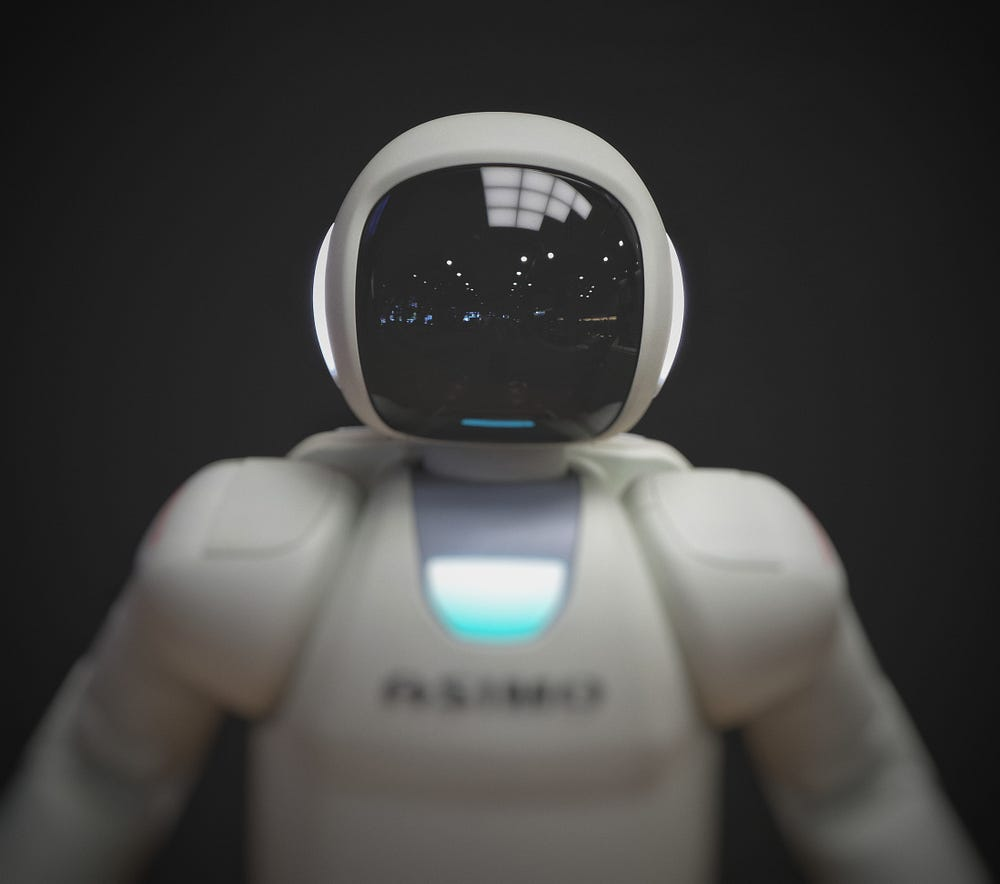I Wrote an AI in 13 Blocks of Scratch

I don’t know about you, but AI to me seem distant, complex, and ever so slightly magical.

You would have reason to think this, if a computer can beat the worlds’ greatest mind at chess or go it would surely have to be incredibly complex, and for the most part, you’d be right, the AlphaGo system is a remarkable feat of computer science. However, it is a common misconception that all machine learning algorithms are like this.

I am not a programmer. Nor I am a computer scientist, and I don’t have a degree in Maths, but I needn’t. AI are ultimately pieces of extreme logicality, and this is something I can do.

I urge you not to click off the article if you aren’t an ML fan. This is as much an exercise in bringing the often cryptic world of computer science into everyone’s domain as anything else.

First I am going to go through the very basic maths behind the model, if you are familiar with the regression technique, feel free to skip to The Code section.

The Maths

My model uses what is known as the regression technique. In simple, this means plotting it on a graph, and extrapolating the correlation.

To link the values, we must draw a line, known as a line of best fit, linking the points. This is shown in the image beside.

Once we have worked the equation of the line, we can calculate the y-value for any x-value, or vice versa.

This line has two characteristics, the gradient, or steepness, and the y-intercept, or where it hits the vertical axis, also shown on the image above.

Now comes the high school maths, this idea can be put into an equation:

Where the gradient is worked out using this equation:

Here you are working out how much y changes for every change in x. For example y might go up by 2 for 1 x goes up by.

Then, with simply addition and subtraction, the y-intercept can be worked out, using real x and y values:

Put this all back into the equation:

So:

Congratulations! Hopefully that wasn’t too strenuous. We now have the basis for our model. Using this, we can input x-values and y-values, and the program will teach itself how to get from x to y.

The Code

For those not familiar with Scratch, every program starts with this block, all it means that when I click start, the program will run:

Next I use up some of my twelve blocks with a little casual conversation.

It is important to note the second block however, this clears the memory of the program, so each time is uses a new set of y-values:

In the next part, I simply ask for the y-value for when x equals one or two, and they are added to my list of y-values:

Here comes the important part! The program uses the values it has just collected to work out the gradient and y-intercept of the graph, using the regression technique we looked at earlier.

Please note that, because the x-values are predetermined, I know their difference will be 1. Therefore, when calculated the gradient, I don’t divide it by the change in x like you normally would.

Finally, plug the variables I have juts calculated into the equation y = mx + c, to get my result for when x = 3:

There we have it! In just a few lines of the simplest programming language in the world we have built an AI!

Now, before I get told off in the comments, this is as basic as an ML model gets. It could be easily replicated with pen and paper, much like we did in The Maths section. However, by definition, it has taken inputs and outputs, without being told how to get from one to the other, and it has worked it out itself. Pretty cool right?

The Extra Code

Now, I know this is technically over the quota from the title, but I couldn’t resist. This extra piece of code allows you to put in your own values:

It works in exactly the same way as the main code, it just uses a user input value instead of the preassigned 3.

Have A Go!

I wrote this on Scratch online, so please follow the link and have a go for yourself:

https://scratch.mit.edu/projects/246490984/

Scratch is more powerful than you might think, I urge you to test you programming skills by trying to build an ML model on it, it is brilliant for getting better at coding because you cannot ‘cheat’ with libraries like in Python.